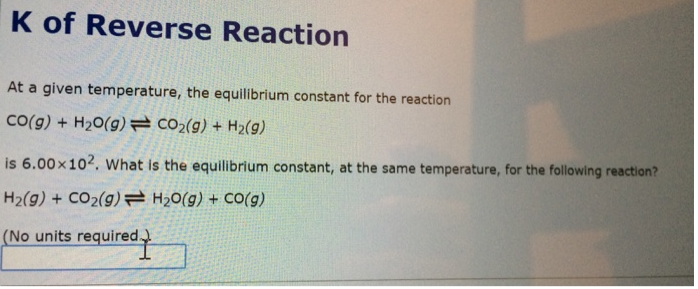# Problem: At a given temperature, the equilibrium constant for the reactionCO(g) + H2O(g) ⇌ CO2(g) + H2(g)is 6.00 x 102. What is the equilibrium constant, at the same temperature, for the following reaction?H2(g) + CO2(g) ⇌ H2O(g) + CO(g)

###### FREE Expert Solution
82% (397 ratings)###### Problem Details

At a given temperature, the equilibrium constant for the reaction

CO(g) + H2O(g) ⇌ CO2(g) + H2(g)

is 6.00 x 102. What is the equilibrium constant, at the same temperature, for the following reaction?

H2(g) + CO2(g) ⇌ H2O(g) + CO(g)